﻿ 舰船导航机器人的自动控制研究
 舰船科学技术2022, Vol. 44Issue (19): 138-141    DOI: 10.3404/j.issn.1672-7649.2022.19.027PDF

1. 天津大学，天津 300072;
2. 北京电子科技职业学院，北京 100176

Research on automatic control of ship navigation robot
LV Shi-xia1,2
1. Tianjin University, Tianjin 300072, China;
2. Beijing Polytechnic, Beijing 100176, China
Abstract: Traditional control methods rely too much on robot motion mechanics, which is prone to yaw on flowing sea level. In order to solve this problem, the automatic control of ship navigation robot is proposed. The edge and contour information of the obstacle are obtained by liDAR identification, and the location information of the destination is determined by constructing a rectangular coordinate system. The structure of the optimal feedback controller is designed, and the optimal control function is constructed. Combined with the linear quadratic optimal control theory, the value of the feedback optimal control function is minimized, and the automatic control law of the ship navigation robot is obtained, so that the system control has dynamic response performance. The dynamic window method was used to control the obstacle avoidance function of the navigation robot, and the kinematics model was constructed. The input deviation signals were converted into points on the domain. The controller was smoothly switched by setting a reasonable threshold, and the motion trajectory equation was derived to realize the automatic control of the navigation direction. The experimental results show that the method can accurately avoid obstacles according to the established route, the Angle deviation is controlled within −5°～5°, and the displacement deviation x, y and z directions are controlled within 0～0.25 m, −1.0～0 m and −0.25～0 m, respectively, which can achieve the purpose of automatic and accurate control.
Key words: navigation robot     automatic control     rectangular coordinate system     optimal feedback controller
0 引　言

1 目的地位置信息确定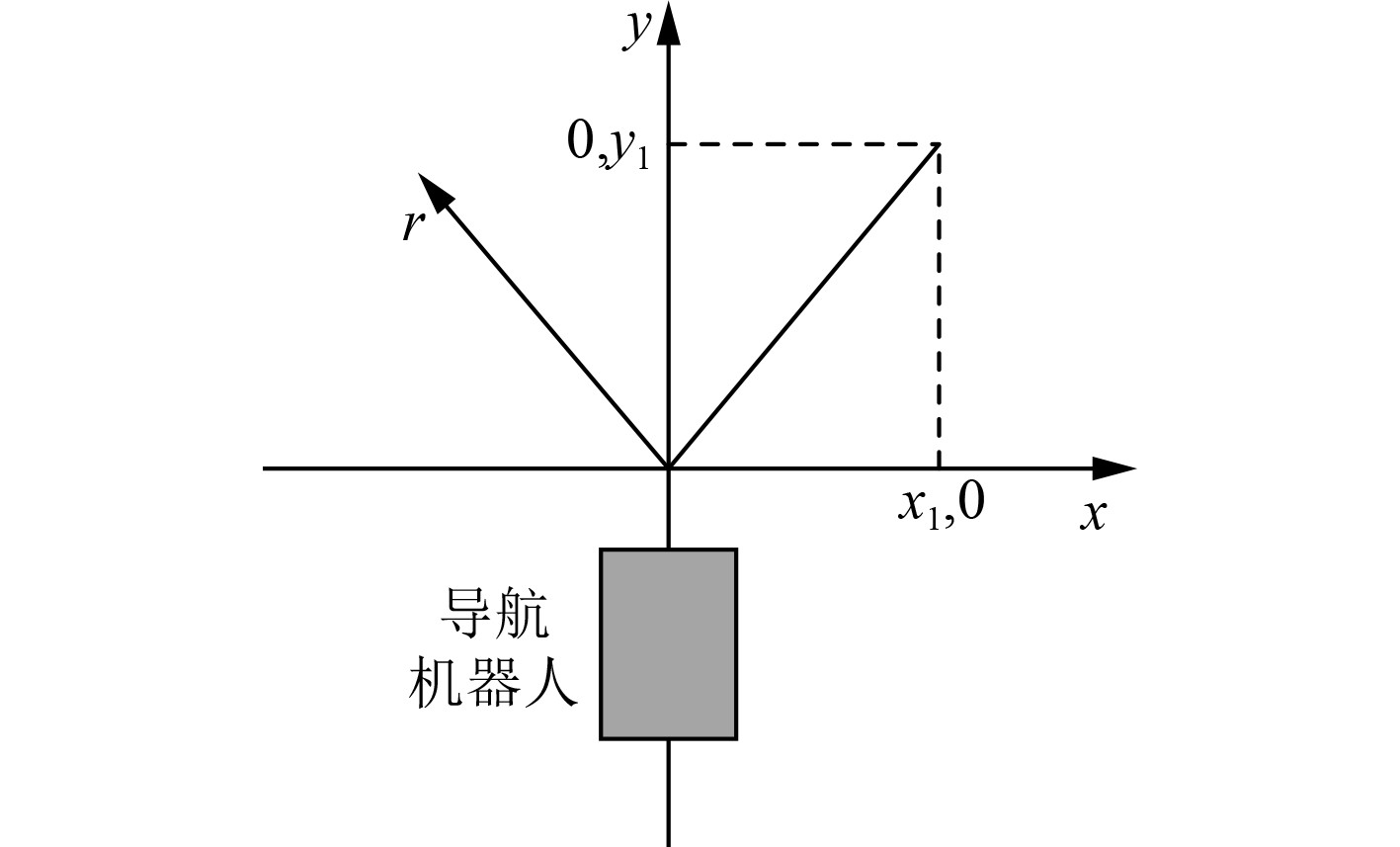图 1 激光扫描直角坐标系 Fig. 1 Laser scanning rectangular coordinate system
2 自动控制方案设计 2.1 反馈控制器设计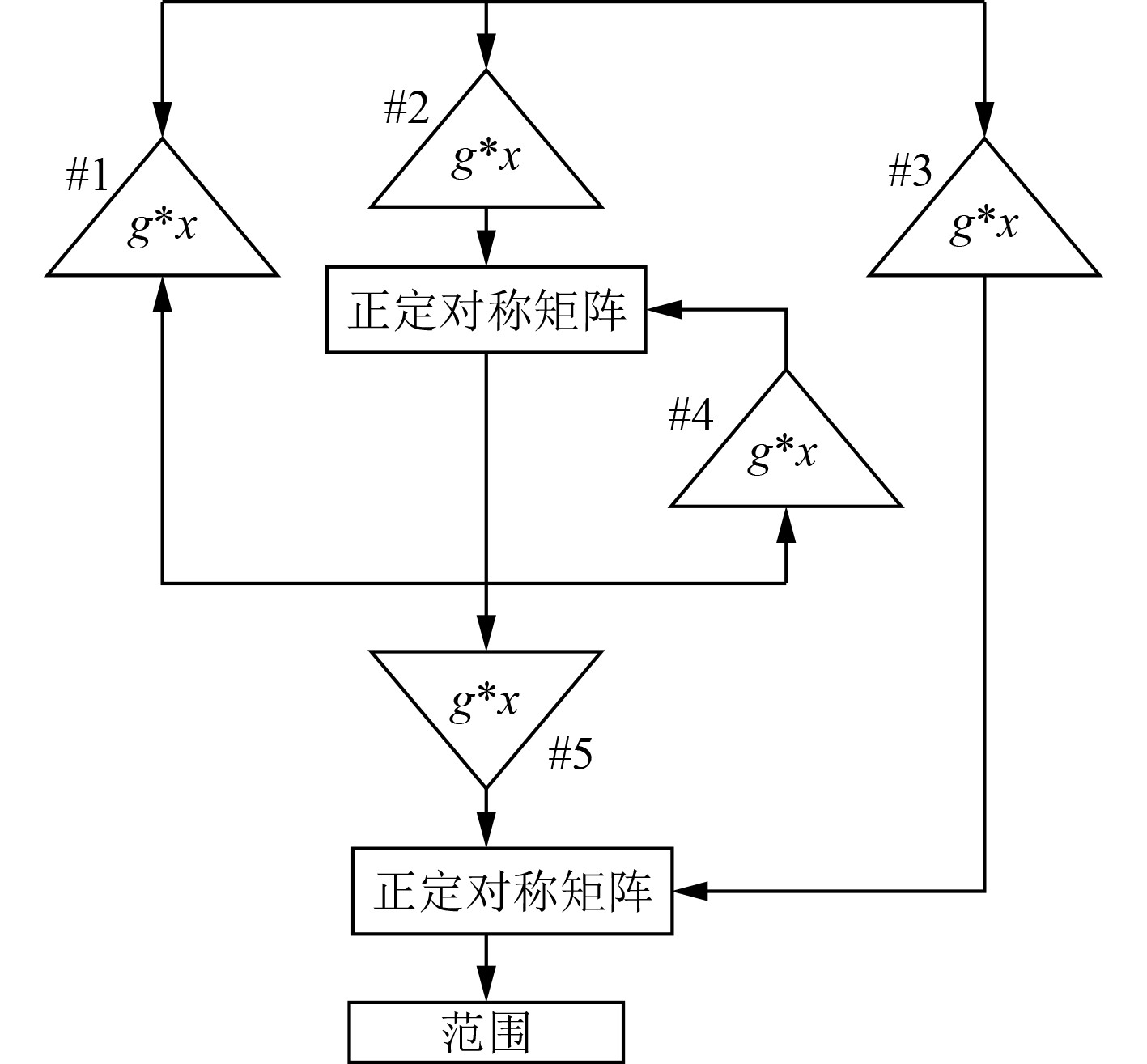图 2 最优反馈控制器结构 Fig. 2 Structure of optimal feedback controller

 $g(l,x)={{\int }}^{\text{}}}_{0}^{\infty }\left[{l}^{\text{T}}(t){\boldsymbol Z}^-(t)l(t)+{x}^{\text{T}}(t){\boldsymbol Z}^+(t)x(t)\right]\text{d}t$ (1)

 $x(t) = - {\boldsymbol{g}} \cdot l(t)。$ (2)

 $\left\{ \begin{gathered} {U_R} = {U_0} - \frac{{\Delta U}}{2} ，\\ {U_L} = {U_0} + \frac{{\Delta U}}{2} 。\\ \end{gathered} \right.$ (3)

 $\Delta U = - \left( {{g_1}\Delta {V_1} + {g_2}\Delta {V_2} + {g_3}\Delta {V_3}} \right)。$ (4)

2.2 导航机器人避障控制

 $\left\{ \begin{gathered} x = {v_x}{{\Delta }}t\cos {\theta _t} - {v_y}{{\Delta }}t\sin {\theta _t}，\\ y = {v_x}{{\Delta }}t\sin {\theta _t} + {v_y}{{\Delta }}t\cos {\theta _t}。\\ \end{gathered} \right.$ (5)

 $\begin{gathered} v \leqslant \sqrt {2d(v) \times {v_b}}，\\ {v_b} \in \left[ {v - {v_c}\Delta t,v + {v_c}\Delta t} \right]。\\ \end{gathered}$ (6)

2.3 偏航控制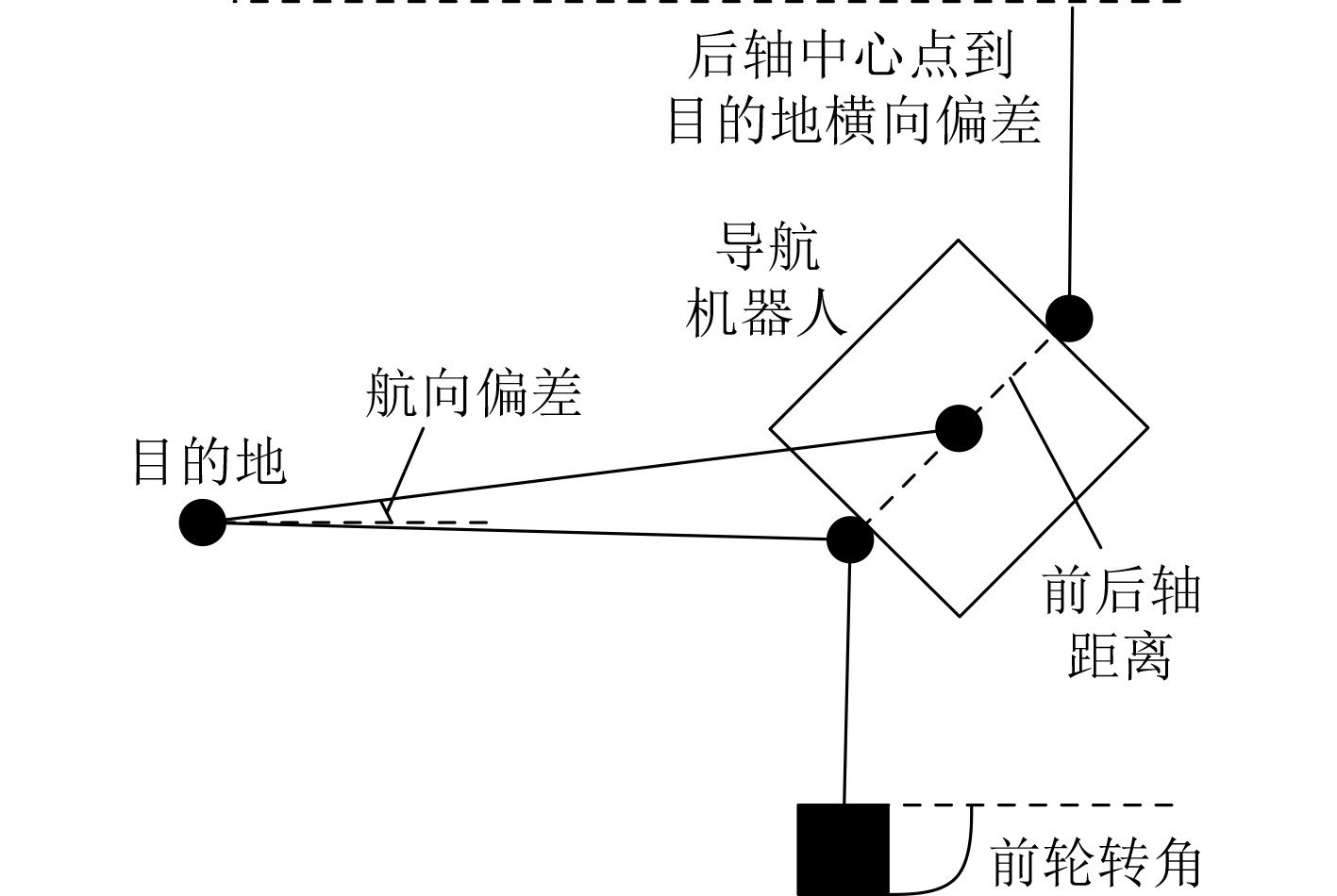图 3 运动学模型 Fig. 3 Kinematic model

 ${m_i} = IT\left( {{\varepsilon _i} \cdot {\lambda _i}} \right)。$ (7)

 ${\varepsilon _i} = \frac{{{m_i}}}{{{\lambda _i}}} 。$ (8)

 $d = v'\sin \alpha 。$ (9)

 $\alpha = \frac{{v'}}{s}\tan \beta。$ (10)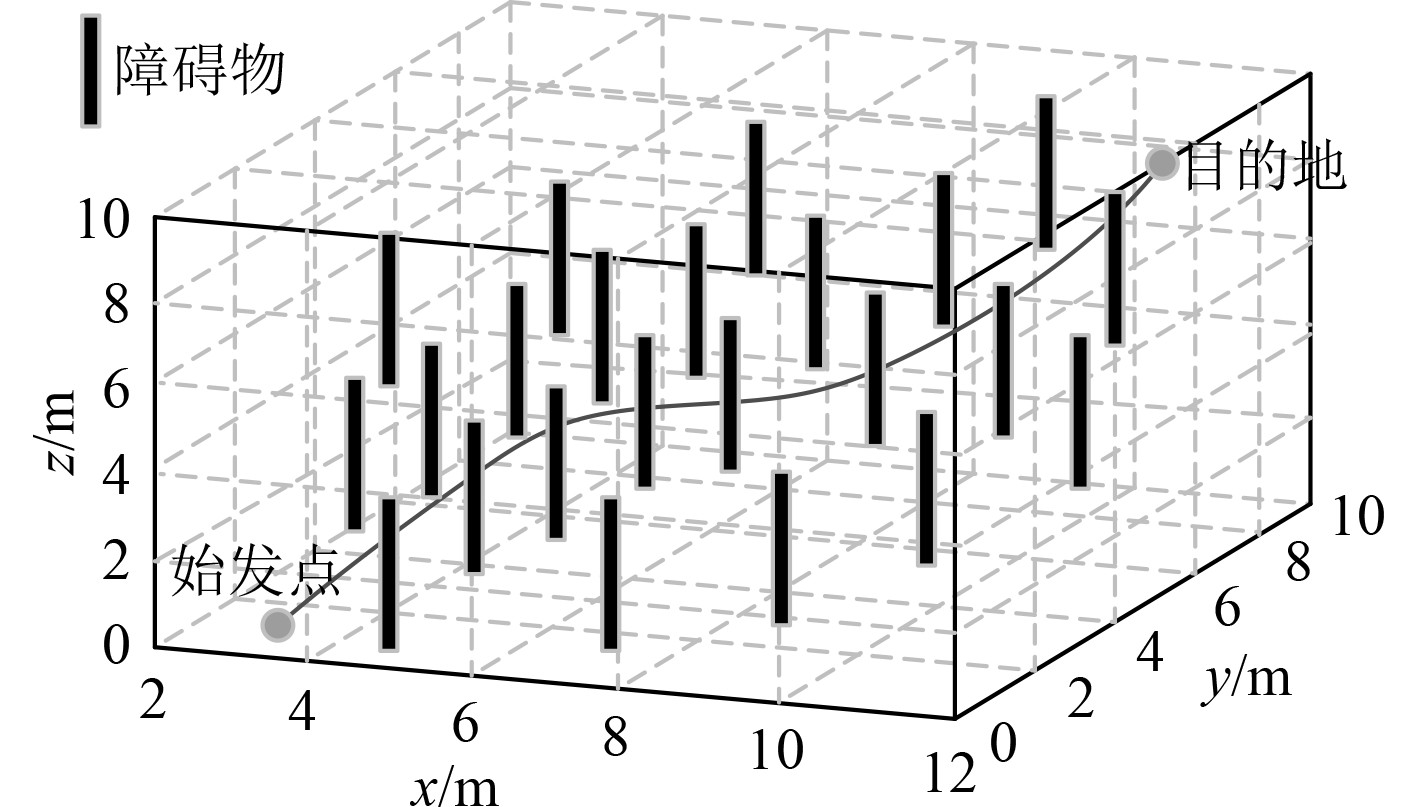图 4 仿真运行结果 Fig. 4 Simulation operation results

3 性能验证性实验 3.1 避障控制测试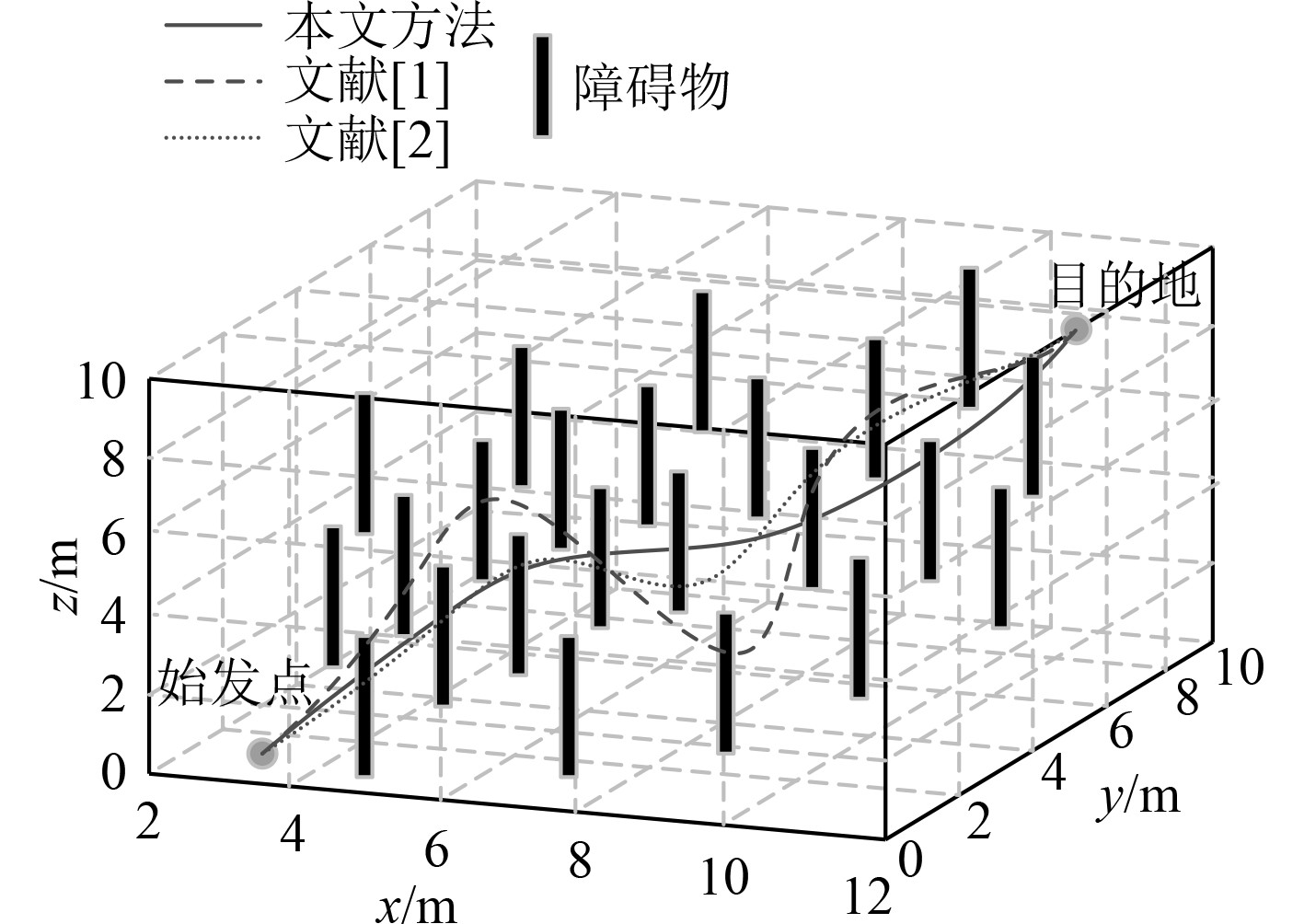图 5 不同方法导航机器人行走路径对比分析 Fig. 5 Comparison and analysis of robot walking paths guided by different methods

3.2 角度与位移偏差验证结果分析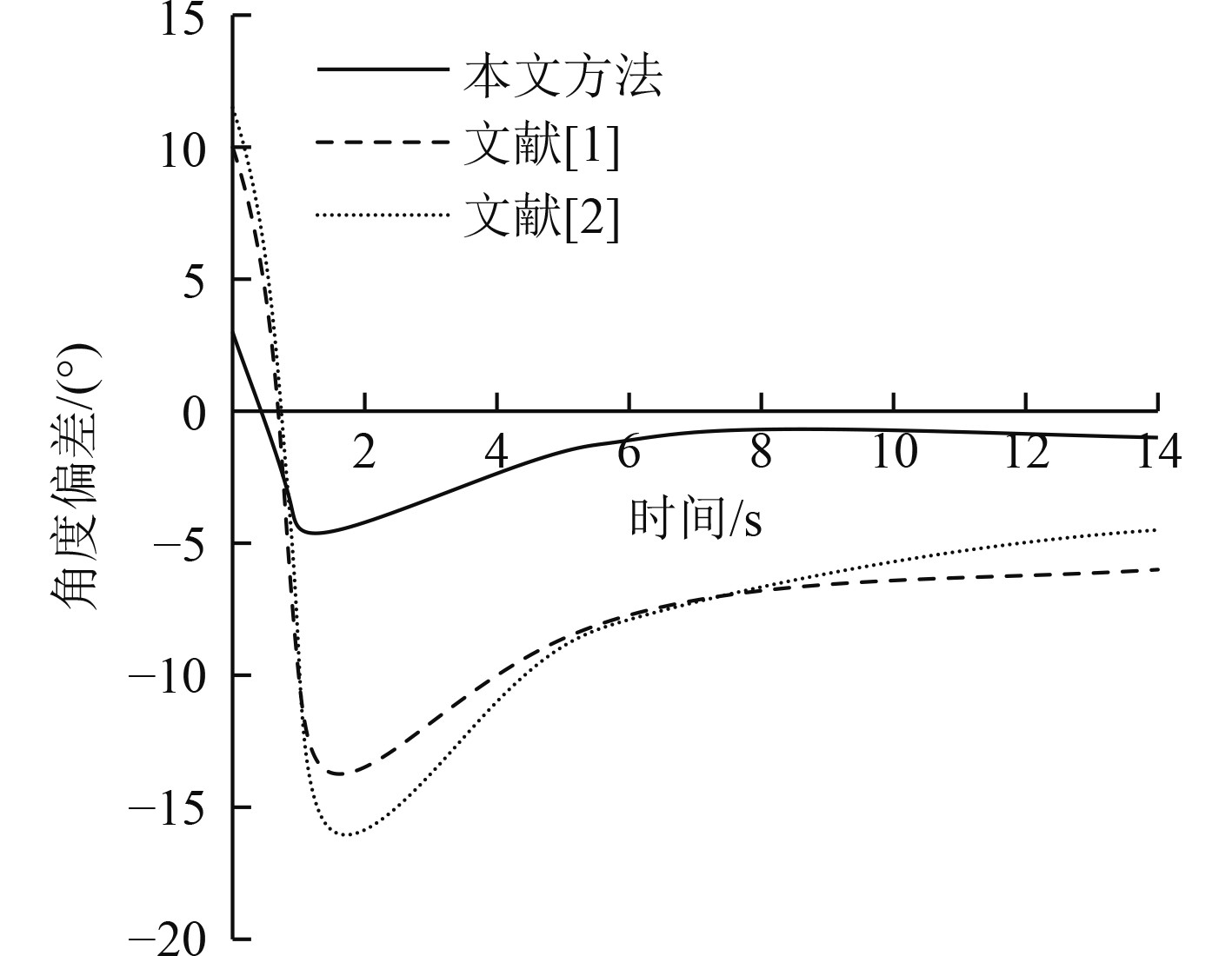图 6 不同方法位移偏差对比分析 Fig. 6 Comparison and analysis of displacement deviation by different methods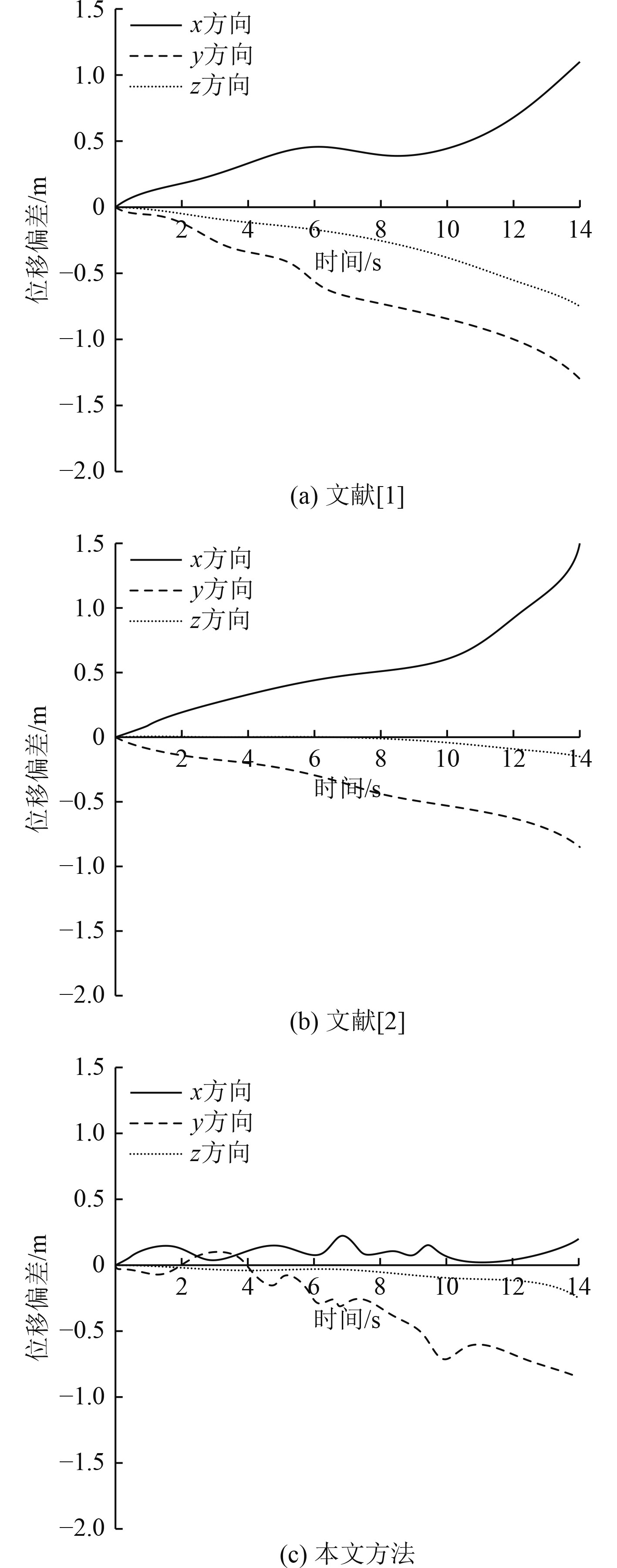图 7 不同方法位移偏差对比分析 Fig. 7 Comparison and analysis of displacement deviation by different methods
4 结　语

  丁坤, 王立, 张经炜, 等. 基于改进导航圆算法的光伏阵列清扫机器人路径跟踪控制[J]. 河海大学学报(自然科学版), 2021, 49(2): 190-196.  李宁. RTK与Lidar组合优化机器人导航控制[J]. 机械设计与制造, 2021(11): 275-278. DOI:10.3969/j.issn.1001-3997.2021.11.062  包加桐, 姚小梅, 缪露, 等. 基于云平台地图的侦察机器人室外自主导航[J]. 控制工程, 2020, 27(6): 941-946.  王钰奉, 陈伟堤, 施祥玲, 等. Delta机器人增广非线性PD控制研究[J]. 组合机床与自动化加工技术, 2021(12): 62-66.  刘冬, 陈飞, 邹强, 等. 基于情景经验与稀疏点云的移动机器人导航[J]. 华中科技大学学报(自然科学版), 2020, 48(9): 25-30.  张玉超, 蒋沅, 代冀阳. 三阶多机器人协同编队动态避障控制[J]. 系统仿真学报, 2022, 34(8): 1762-1774. DOI:10.16182/j.issn1004731x.joss.21-0322  李航, 王朝耀. 仓库搬运机器人导航运动控制与NURBS轨迹规划[J]. 机械设计与制造, 2020(7): 290-295. DOI:10.3969/j.issn.1001-3997.2020.07.066  李峰, 岳云, 张跃进, 等. 基于北斗导航的机器人弹道曲线航道导航系统[J]. 弹道学报, 2021, 33(2): 47-54.